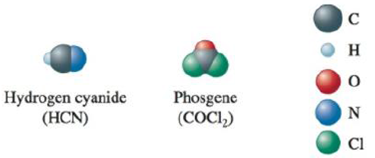# The space-filling models of hydrogen cyanide and phosgene are shown below. Use the localized electron model to describe the bonding in hydrogen cyanide and phosgene.### Chemistry: An Atoms First Approach

2nd Edition
Steven S. Zumdahl + 1 other
Publisher: Cengage Learning
ISBN: 9781305079243

#### Solutions

Chapter
Section### Chemistry: An Atoms First Approach

2nd Edition
Steven S. Zumdahl + 1 other
Publisher: Cengage Learning
ISBN: 9781305079243
Chapter 4, Problem 44E
Textbook Problem
12 views

## The space-filling models of hydrogen cyanide and phosgene are shown below.Use the localized electron model to describe the bonding in hydrogen cyanide and phosgene.

Interpretation Introduction

Interpretation: The bonding in the given compounds, hydrogen cyanide and phosgene, is to be described using the localized electron model.

Concept introduction: The valence bond theory is based on the assumption that all the bonds present between two atoms are localized bonds formed by the donation of an electron from each atom. The localized electron model consists of the following,

• The Lewis structure is drawn.
• The arrangement of the electron pairs is determined.
• The required hybrid orbitals are specified.

To determine: Bonding in the given compounds, on the basis of the localized electron model.

### Explanation of Solution

The electronic configuration of the elements present in hydrogen cyanide is,

H=1s1C=1s22s22p2N=1s22s22p3

Hydrogen shows the presence of only one electron. The valence electrons present in carbon is four and in nitrogen is five.

The total number of valence electrons in (HCN) is 1e+(1×4)e+5e=10e .

The Lewis structure of the given compound is,

Figure 1

For this compound,

• In the given compound, the triple bond present is counted as an effective pair that requires a linear arrangement. This corresponds to the sp hybridization. Two unchanged p orbitals are present.
• One of the sp orbitals present, oppositely oriented, is used in the formation of a σ bond bond with the hydrogen atom.
• The other sp orbital present shows overlapping with the sp orbital of nitrogen leading to the formation of a σ bond.
• The presence of another sp orbital is observed, present perpendicular to the plane of the bonding orbitals.
• The orbitals that do not participate in the hybridization partially combine leading to the π bonding between the carbon and the nitrogen atom

### Still sussing out bartleby?

Check out a sample textbook solution.

See a sample solution

#### The Solution to Your Study Problems

Bartleby provides explanations to thousands of textbook problems written by our experts, many with advanced degrees!

Get Started

Find more solutions based on key concepts
Magnesium a. assists in the operation of enzymes. b. is needed for the release and use of energy. c. is critica...

Nutrition: Concepts and Controversies - Standalone book (MindTap Course List)

Describe the respiratory mechanism for controlling blood pH.

Chemistry for Today: General, Organic, and Biochemistry

When high-energy charged particles move through a transparent medium with a speed greater than the speed of lig...

Physics for Scientists and Engineers, Technology Update (No access codes included)